UPSC  >  Important Formulas: Clocks

# Important Formulas: Clocks - Notes | Study CSAT Preparation for UPSC CSE - UPSC

 Table of contentsPoints to RememberSolved Problems1 Crore+ students have signed up on EduRev. Have you?

Points to Remember

1. Minute Spaces: The face or dial of the clock is a circle whose circumference is divided into 60 equal parts, named minute spaces.
2. The hour hand and minute hand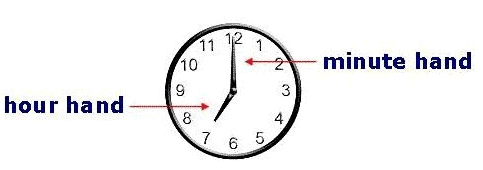A clock has two hands. The smaller hand is called the hour hand or shorthand and the larger one is called the minute hand or longhand.
3. In 60 minutes, the minute hand gains 55-minute spaces over the hour hand.
(In 60 minutes, the hour hand will move 5-minute spaces while the minute hand will move 60-minute spaces. In effect the space gain of minute hand with respect to hour hand will be 60 - 5 = 55 minutes.)
4. Both the hands of a clock coincide once every hour.
Example: Between 11 and 1'o clock, hands are together as shown in the adjacent figure.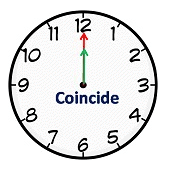5. The hands of a clock are in the same straight line when they are coincident or opposite to each other.
• If two hands are in opposite directions. (180° apart), then they are 30 min spaces apart. This happens once in 1 h. In a period of 12 hours, both hands are in opposite direction 11 times and in a day both hands are in opposite direction 22 times.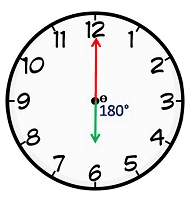6. When the two hands of a clock are at right angles, they are 15-minute spaces apart.
This happens twice in 1 h. In a period of 12 h, the hands are at a right angle 22 times (2 common positions) and in a day both hands are at a right angle 44 times.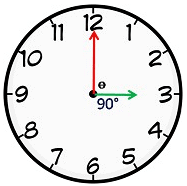7. When the hands of a clock are in opposite directions, they are 30-minute spaces apart.
8. Angle traced by hour hand in 12 hrs = 360°
9. Angle traced by minute hand in 60 min. = 360°.
Question for Important Formulas: Clocks
Try yourself:An accurate clock shows 8 o'clock in the morning. Through how many degrees will the hour hand rotate when the clock shows 2 o'clock in the afternoon?
10. If a watch or a clock indicates 9.15, when the correct time is 9, it is said to be 15 minutes too fast.
11. If a watch or a clock indicates 8.45, when the correct time is 9, it is said to be 15 minutes too slow.
12. The hands of a clock will be in a straight line but opposite in direction, 22 times in a day.
13. The hands of a clock coincide 22 times in a day.
14. The hands of a clock are straight 44 times in a day.
15. The hands of a clock are at right angles 44 times a day.
16. The two hands of a clock will be together between H and (H+1) o'clock at
(60 H/ 11) minutes past H o'clock.
17. The two hands of a clock will be in the same straight line but not together between H and (H+1) o'clock at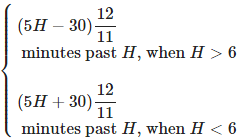18. The angle between the hands of a clock
(i) When the minute hand is behind the hour hand, the angle between the two hands at M minutes past H 'o clock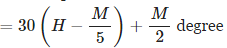(ii) When the minute hand is ahead of the hour hand, the angle between the two hands at M minutes past H 'o clock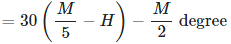Question for Important Formulas: Clocks
Try yourself:A clock starts at noon. By 10 minutes past 5, the hour hand has turned through:
19. The two hands of the clock will be at right angles between H and (H+1) o'clock at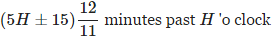If the minute hand of a clock overtakes the hour hand at intervals of M minutes of the correct time, the clock gains or loses in a day by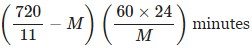Question for Important Formulas: Clocks
Try yourself:An accurate clock shows 7 a.m. Through how many degrees will the hour hand rotate when the clock shows 1 p.m.?
20. Between H and (H+1) o'clock, the two hands of a clock are M minutes apart at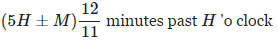Solved Problems

Example 1: At what time between 4 and 5, the minute hand will be 2 minutes spaces ahead of the hour hand?

At 4 O'clock, the two hands are 20 min spaces apart.

In this case, the min hand will have to gain (20 + 2) i.e. 22 – minute spaces.

So, 22 – minute spaces will be gained in (60/55) × 22 = 24 min.

Example 2: The minute hand of a clock overtakes the hour hand at intervals of 65 minutes of correct time. How much does the clock lose or gain in 12 hours?

In a correct clock, the minute hand and hour hand should meet after every 65 5/11 min.

But we know that they are meeting after every 65 minutes.

So, the gain in 65 minutes is 5/11 minutes.

Gain in 12 hours = (120 × 60/65) × (5/11) = 720/143 = 5 5/143 min.

Question for Important Formulas: Clocks
Try yourself:At what time between 5.30 and 6 will the hands of a clock be at right angles?

Example 3: A clock is set right at 10 am. The clock gains 5 minutes in 12 hours. What will be the true time when the clock indicates 3 pm on the next day?

Time from 10 am to 3 pm on the following day is 29 hrs.

Now, 12 hrs 5 min i.e. 145/12 hrs of this clock = 12 hours of correct clock.

So, 29 hours of this clock is (29 × 12 × 12)/145 = 144/5 = 28 hours 48 minutes.

So, the time is 12 minutes before 3 pm.

Example 4: The minute hand of a clock overtakes the hour band at interval of 60 mins. How much a day does the clock gain or lose?

In a correct clock, minute hand gains 55 minute spaces over the hour hand in 60 minutes.

Therefore, 60 min spaces are gained in (60 × 60)/55 = 720/11 minutes.

In other words, minute hand overtakes hour hand in every 720/11 minutes.

In the example given, minute hand overtakes hour band in 60 minutes.

Therefore, gain in 60 minute = (720/11) - 60 = 60/11 minutes.

Gain in 24 hours = (60/11) × 24 = 1440/11 min

gain/loss =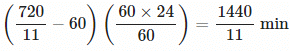Since the sign is +ve, clock gains 1440/11 min in a day.

Question for Important Formulas: Clocks
Try yourself:How many times in a day, the hands of a clock are straight?

Example 5: The minute hand of a clock overtakes the hour band at an interval of 66 mins. How much a day does the clock gain or lose?

As we have seen previously, minute hand overtakes hour hand in every 720/11 minute.

In this example, minute hand overtakes hour band in 66 minutes

Therefore, loss in 66 minute = 66 - 720/11 = 6/11 minutes

Loss in 24 hours = 6/11 × 1/66 × 66 × 24 = 1440/121 min

gain/loss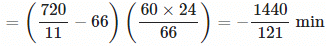Since the sign is -ve, the clock loses 1440/121 min a day.

The document Important Formulas: Clocks - Notes | Study CSAT Preparation for UPSC CSE - UPSC is a part of the UPSC Course CSAT Preparation for UPSC CSE.
All you need of UPSC at this link: UPSC

## CSAT Preparation for UPSC CSE

72 videos|64 docs|92 tests
 Use Code STAYHOME200 and get INR 200 additional OFF

## CSAT Preparation for UPSC CSE

72 videos|64 docs|92 tests

### How to Prepare for UPSC

Read our guide to prepare for UPSC which is created by Toppers & the best Teachers

Track your progress, build streaks, highlight & save important lessons and more!

,

,

,

,

,

,

,

,

,

,

,

,

,

,

,

,

,

,

,

,

,

;